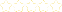• > Jeff Franz
• > Slope and Linear Graphing
+# Slope and Linear Graphing

Owner: Jeff Franz
##### Description:
Common Core Math 8.EE5, 8.EE.6

### Your Quiz Points in this Playlist

Total Possible
0
8 Tutorials in this Playlist

#### Finding Slope from Unit Rates

Author: Jeff Franz
Rating:(37)
This tutorial has a quiz
•• -

#### Finding Slope from Two Points

Author: Jeff Franz
Rating:(0)

#### Finding Slope from a Line

Author: Jeff Franz
Rating:(1)

#### Using Similar Triangles to find Slope of a Line

Author: Jeff Franz
Rating:(3)

#### Finding Slope from a Table

Author: Jeff Franz
Rating:(1)

#### Finding Slope from an Equation

Author: Jeff Franz
Rating:(0)

#### Writing Linear Equations (y = mx) from a Graph

Author: Jeff Franz
Rating:(2)

#### Writing Linear Equations (y = mx + b) from a Graph

Author: Jeff Franz
Rating:(1)